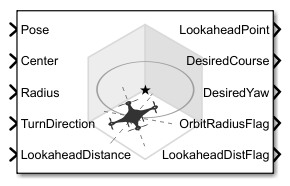# Orbit Follower

Orbit location of interest using UAV

Since R2019a

•Libraries:
UAV Toolbox / Algorithms

## Description

The Orbit Follower block generates course and yaw controls for following a circular orbit around a location of interest based on the current pose of the unmanned aerial vehicle (UAV). Select a UAV type of fixed-wing or multirotor UAVs. You can specify any orbit center location, orbit radius, and turn direction. A lookahead distance, LookaheadDistance, is used for tuning the path tracking and generating the LookaheadPoint output.

## Ports

### Input

expand all

Current UAV pose, specified as an `[x y z course]` vector. `[x y z]` is the position of the UAV in NED coordinates (north-east-down) specified in meters. `course` is the angle between ground velocity and north direction in radians per second.

Example: `[1,1,-10,pi/4]`

Data Types: `single` | `double`

Center of orbit, specified as an `[x y z]` vector. ```[x y z]``` is the orbit center position in NED coordinates (north-east-down) specified in meters.

Example: `[5,5,-10]`

Data Types: `single` | `double`

Radius of orbit, specified as a positive scalar in meters.

Example: `5`

Data Types: `single` | `double`

Direction of orbit, specified as a scalar. Positive values indicate a clockwise turn as viewed from above. Negative values indicate a counter-clockwise turn. A value of `0` automatically determines the value based on the input to Pose.

Example: `-1`

Data Types: `single` | `double`

Lookahead distance for tracking the orbit, specified as a positive scalar. Tuning this value helps adjust how tightly the UAV follows the orbit circle. Smaller values improve tracking, but can lead to oscillations in the path.

Example: `2`

Data Types: `single` | `double`

Reset for counting turns, specified as a numeric signal. Any rising signal triggers a reset of the NumTurns output.

Example: `2`

#### Dependencies

To enable this input, select `rising` for External reset.

Data Types: `single` | `double`

### Output

expand all

Lookahead point on path, returned as an `[x y z]` position vector in meters.

Data Types: `double`

Desired course, returned as numeric scalar in radians in the range of `[-pi, pi]`. The UAV course is the angle of direction of the velocity vector relative to north measured in radians. For fixed-wing type UAV, the values of desired course and desired yaw are equal.

Data Types: `double`

Desired yaw, returned as numeric scalar in radians in the range of ```[-pi, pi]```. The UAV yaw is the forward direction of the UAV (regardless of the velocity vector) relative to north measured in radians. For fixed-wing type UAV, the values of desired course and desired yaw are equal.

Data Types: `double`

Orbit radius flag, returned as `0` or `1`. `0` indicates orbit radius is not saturated, `1` indicates orbit radius is saturated to minimum orbit radius value specified.

Data Types: `uint8`

Lookahead distance flag, returned as `0` or `1`. `0` indicates lookahead distance is not saturated, `1` indicates lookahead distance is saturated to minimum lookahead distance value specified.

Data Types: `uint8`

Cross track error from UAV position to path, returned as a positive numeric scalar in meters. The error measures the perpendicular distance from the UAV position to the closest point on the path.

#### Dependencies

This port is only visible if Show CrossTrackError output port is checked.

Data Types: `double`

Number of times the UAV has completed the orbit, returned as a numeric scalar. As the UAV circles the center point, this value increases or decreases based on the specified Turn Direction. Decimal values indicate partial completion of a circle. If the UAV cross track error exceeds the lookahead distance, the number of turns is not updated.

NumTurns is reset whenever Center, Radius, or TurnDirection are changed. You can also use the ResetNumTurns input.

#### Dependencies

This port is only visible if Show NumTurns output port is checked.

## Parameters

expand all

Type of UAV, specified as either `fixed-wing` or `multirotor`.

This parameter is non-tunable.

Minimum orbit radius, specified as a positive numeric scalar in meters.

When input to the orbit Radius port is less than the minimum orbit radius, the OrbitRadiusFlag is returned as `1` and the orbit radius value is specified as the value of minimum orbit radius.

This parameter is non-tunable.

Minimum lookahead distance, specified as a positive numeric scalar in meters.

When input to the LookaheadDistance port is less than the minimum lookahead distance, the LookaheadDistFlag is returned as `1` and the lookahead distance value is specified as the value of minimum lookahead distance.

This parameter is non-tunable.

Select `rising` to enable the ResetNumTurns block input.

This parameter is non-tunable.

Output cross track error from the CrossTrackError port.

This parameter is non-tunable.

Output UAV waypoint status from the Status port.

This parameter is non-tunable.

## Version History

Introduced in R2019a